# A steel

A steel hammer with a weight of 0.5 kg hits the nail at a speed of 3 m/s. How deep the nail penetrates the wood if the hammer acts on the nail with an average force of 50N.

x =  45 mm

### Step-by-step explanation: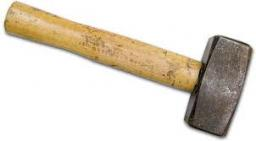Did you find an error or inaccuracy? Feel free to write us. Thank you!Tips to related online calculators

## Related math problems and questions:

• A hammer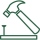A hammer weighing 500 g hits the nail at a speed of 3 m/s. What is the average force acting on the nail, which penetrates the board to a depth of 5 cm?
• Shot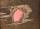Shot with a mass 43 g flying at 256 m/s penetrates into the wood to a depth 25 cm. What is the average force of resistance of wood?
• CarAt what horizontal distance reaches the car weight m = 753 kg speed v = 74 km/h when the car engine develops a tensile force F = 3061 N. (Neglect resistance of the environment.)
• The carThe car weight 1280 kg, increased its speed from 7.3 m/s to 63 km/h on a track of 37.2 m. What force did the car engine have to exert?
• Positional energyWhat velocity in km/h must a body weighing 60 kg have for its kinetic energy to be the same as its positional energy at the height 50 m?
• Elevator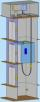In homes with more floor elevators are used. For passenger transport, the most commonly used traction elevator counterweight. The top of the shaft engine room with the engine. The car is suspended on a rope, which is guided up over two pulleys to the coun
• Center of gravityThe mass points are distributed in space as follows - specify by coordinates and weight. Find the center of gravity of the mass points system: A1 [1; -20; 3] m1 = 46 kg A2 [-20; 2; 9] m2 = 81 kg A3 [9
• Hollow sphereThe steel hollow sphere floats on the water plunged into half its volume. Determine the outer radius of the sphere and wall thickness, if you know that the weight of the sphere is 0.5 kg and the density of steel is 7850 kg/m3
• Inclined plane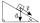1. How much work W we have to do to pull a body weighing 200 kg along an inclined plane with a length of 4 m to a total height of 1.5 m. 2. Find the force we need to exert to do this if we neglect frictional resistance. 3. Find the force we would need if
• Cuboid 5Calculate the mass of the cuboid with dimensions of 12 cm; 0.8 dm and 100 mm made from spruce wood (density = 550 kg/m3).
• Wood cuboidWhat is the weight of the wood cuboid 15 cm, 20 cm, 3 m if 1 m3 wood weighs 800 kg?
• The swing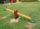To swing the two girls. Aneta weight 45 kg and Simon 35 kg weight. How far should sit Simon from the middle of swing so it is balanced, if we know that Aneta is sitting at distance 1,5m? How far are girls sitting apart?
• Dynamometer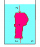What is the volume of a body that stretches a dynamometer in air, on which it is suspended by a force of 2.5 N, and if it is immersed in alcohol with a density of 800 kg/m ^ 3, does it tension the dynamometer with a force of 1.3 N?
• The bridge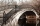A vehicle weighing 5,800 kg passes 41 km/h on an arched bridge with a radius of curvature of 62 m. What force is pushing the car onto the bridge as it passes through the center? What is the maximum speed it can cross over the center of the bridge so that
• Hydrostatic forceWhat is the hydrostatic force applied to an area of 30 cm2 in the water at a depth of 20 m? (Water density is 1000 kg/m3)
• Free fall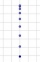The elevator car weighing 720 kg is torn off at a height of 125 m and begins to fall in a free fall. How much kinetic energy does the elevator car have when it hits the ground floor of the building, when the free fall of the car lasted 5 s?
• Eiffel TowerEiffel Tower in Paris is 300 meters high and is made of steel. Its weight is 8000 tons. How tall is the tower model made ​​of the same material if it weighs is 2.4 kg?